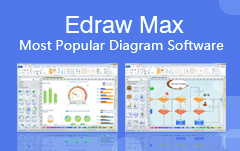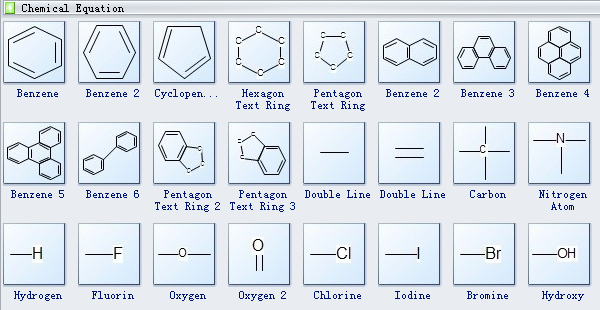Home
• Products
• Pricing
• Office Efficiency
• Project Management
• Graphic Design
• Engineering
• Education
• Special Industry
• OthersCreating chemistry equation with free templates and examples. Chemistry equation has never been easier.

### Chemistry Equation

A chemical equation is the symbolic representation of a chemical reaction. In the chemistry equation, the reactant entities are given on the left hand side and the product entities are given on the right hand side. The first chemical equation was diagrammed by Jean Beguin in 1615. A chemical equation can be used to describe a physical reaction, which involves a change of states, for example, melting, sublimation, evaporation, etc.
Chemical equations diagrams show clearly the reactants and products of a chemical reaction. Therefore, chemical equations diagrams are very helpful in communicating how elements are arranged in a compound.

### Chemistry Equation Software

Edraw Max is a vector-based chemistry equation drawing software allowing you both hand-draw and drag-and-drop pre-made shapes. It includes lots of scientific illustration templates and examples which make it easy to draw Chemistry Equation, Molecular Formulae, Structural Formulae, Benzene Diagram, Molecular Model Diagram, Laboratory Equipment and Chemical sketch illustrations.

### Chemistry Equation Symbols

Edraw software offers you plenty of chemical equipment, benzene, cyclopentane, hexagon text ring, benzene, pentagon text ring, double line, carbon, nitrogen atom, hydrogen, fluorin, oxygen, chlorine, iodine, bromine, hydroxyl, etc.### Chemistry Equation Diagram Examples

A chemistry equation template is a great helper for your own designs, being the second-to-none option for its simplicity and efficiency.

## Get Started! You Will Love This Easy-To-Use Diagram Software.

Edraw Max is perfect not only for professional-looking flowcharts, organizational charts, mind maps, but also network diagrams, floor plans, workflows, fashion designs, UML diagrams, electrical diagrams, science illustration, charts and graphs... and that is just the beginning!